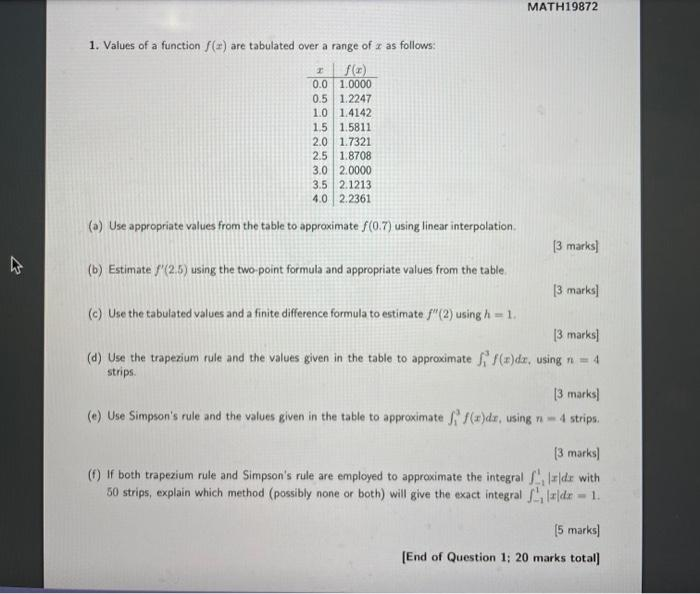# (Solved): 1. Values of a function $$f(x)$$ are tabulated over a range of $$x$$ as follows: (a) Use approp ...1. Values of a function $$f(x)$$ are tabulated over a range of $$x$$ as follows: (a) Use appropriate values from the table to approximate $$f(0.7)$$ using linear interpolation. [3 marks] (b) Estimate $$f^{\prime}(2.5)$$ using the two-point formula and appropriate values from the table. [3 marks] (c) Use the tabulated values and a finite difference formula to estimate $$f^{\prime \prime}(2)$$ using $$h=1$$. [3 marks] (d) Use the trapezium rule and the values given in the table to approximate $$\int_{1}^{3} f(x) d x$$, using $$n=4$$ strips. [3 marks] (e) Use Simpson's rule and the values given in the table to approximate $$\int_{1}^{3} f(x) d x$$, using $$n=4$$ strips. [3 marks] (f) If both trapezium rule and Simpson's rule are employed to approximate the integral $$\int_{-1}^{1}|x| d x$$ with 50 strips, explain which method (possibly none or both) will give the exact integral $$\int_{-1}^{1}|x| d x=1$$. [5 marks] [End of Question 1; 20 marks total]

We have an Answer from Expert